Courses

# Test: Number Systems - 2

## 25 Questions MCQ Test Quantitative Aptitude for GMAT | Test: Number Systems - 2

Description
This mock test of Test: Number Systems - 2 for Class 9 helps you for every Class 9 entrance exam. This contains 25 Multiple Choice Questions for Class 9 Test: Number Systems - 2 (mcq) to study with solutions a complete question bank. The solved questions answers in this Test: Number Systems - 2 quiz give you a good mix of easy questions and tough questions. Class 9 students definitely take this Test: Number Systems - 2 exercise for a better result in the exam. You can find other Test: Number Systems - 2 extra questions, long questions & short questions for Class 9 on EduRev as well by searching above.
QUESTION: 1

### How many prime number are there between 0 and 30 :-

Solution:

There are 10 prime numbers between 0 and 30 which are: 2,3,5,7,11,13,17,19,23,29

QUESTION: 2

### Two irrational numbers between 2 and 2.5 are :-

Solution:

Irrational number between 2 and 2.5 = √2*2.5 = √5

As it is clear now that,

2 < √5 < 2.5

We know that √5 is an irrational number.

So, √5 is the required irrational number between 2 and 2.5.

QUESTION: 3

### The exponential form of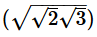is :-

Solution:

(√2×√3)½ = (2×3)½×½ = (6)¼

QUESTION: 4

The rational form of –25.6875 is :-

Solution: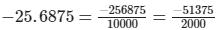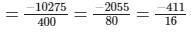QUESTION: 5

The rational form of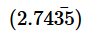is :-

Solution:

As the bar is under two digits multiply with 100

100x = 2.7435*100

100x = 274.35

now subtract with x terms

that gives

99x = 274.35 - 2.7435

99 x = 271.60

x = 271.60/99

x = 2716/9900

QUESTION: 6

The value of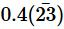is :-

Solution:
QUESTION: 7

Which of the following is not a rational number :-

Solution:

√4 = 2, √9 = 3, √16 = 4 but √2 = 1.414 it is a non-terminating and non-repeating number and such numbers are called irrational numbers. So, √2 is not a rational number.

QUESTION: 8

(2√3 +√3) is equal to

Solution:

(2√3 +√3) = 3√3       ( By addition of like terms )

So option C is correct answer.

QUESTION: 9

The number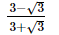is :-

Solution:

If this number is rationalised then 3-√3×3-√3/3+√3×3-√3
=(3-√3)2/3^2+√32
=9+3-6√3/6
=12-6√3/6
=2-√3 this is an irrational number.

QUESTION: 10

If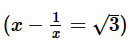then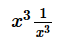equals :-

Solution:
QUESTION: 11

The value of 5.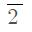:-

Solution:
QUESTION: 12

The sum of the digits of a number is subtracted from the number, the resulting number is always divisible by which of the following numbers?

Solution:

The resulting number is always divisible by 9. e.g., Consider 47. Sum of its digits is 4+7=11 So,47−11=36 which is exactly divisible by 9

QUESTION: 13

The value of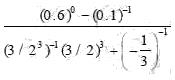is  :-

Solution:
QUESTION: 14

If 2x = 4y = 8z and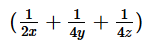= 4, then the value of x is :-

Solution:
QUESTION: 15

If 9x-1 = 32x-1 – 486 then the value of x is :-

Solution:
QUESTION: 16

If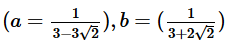then the value of a2 +b2 is :-

Solution:

a = 1/ (3-2√2)

b =  1/ ( 3+2√2)

∴ ab =  1/(3-2√2) * 1/ (3+2√2)

=>  1/ (9-8)

=> 1

We know;

(a+b)2 = a2 + b+ 2ab

And, a2+ b2 = (a+b)2 - 2ab

=> [ 1/(3-2√2) + 1/(3+2√2) ]2 -2*1

=> [3+2√2+3-2√2 / 1]^2 -2

=> (6)2 - 2

=>  36 - 2
=>  34

QUESTION: 17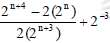is equal to :-

Solution:
QUESTION: 18

If 22x-y = 32 and 2x+y = 16 then x2 + y2 :-

Solution: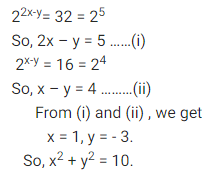QUESTION: 19

The value of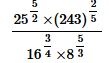is :-

Solution:
QUESTION: 20

The value of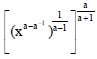Solution:
QUESTION: 21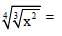Solution:

[(x)^2]1/3]1/4

= [(x2)1/12]

= x1/6

QUESTION: 22

The value of 4√3 – 3 √12 + 2 √75 on simplifying is :-

Solution: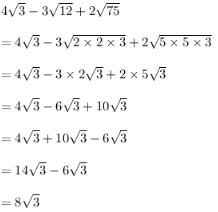QUESTION: 23

If √3 = 1.732, √5 = 2.236, then the value of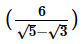is :-

Solution:
QUESTION: 24

The product of 4 √6 and 3√24 is :-

Solution:

4√6*3√24=4√6*3√2*√2*√*2*√3

=4√6*6√6

4*6*√6*√6=4*6*6=24*6=144

QUESTION: 25

If a =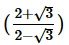, b =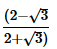, then the value of a + b is :-

Solution: